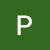# Gradient descent in python with example

If we have any function and we want find the extremum of that function whether it is minima or maxima we use gradient descent optimization technique . so in this blog we will see gradient descent and its variants in python. this blog assumes that you already know little bit about derivative and linear regression

Let we have function y=f(x)=x**2+3

Now here we can see minimum value occurs when x=0 . now if we want to find minimum value of any function we take derivative of it with respect to variable(x) and then equate it to 0 .

Y= x**2…

# Computational Complexity of ML algorithms

Time and space complexity plays very important role while selecting machine learning algorithm

Space complexity: space complexity of an algorithm denotes the total space used or needed by the algorithm for its working , for various input size. In simple words space it requires to complete the task.

Time complexity: The time complexity is the number of operations on algorithm perform to complete its task with respect to input size. In simple words time it requires to complete the task.

In this blog we will see train and run time complexity of ml algorithms .

# k-nearest neighbors (KNN)

Assumption : the knn algorithm…data scientist